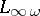# Transfer principle

(diff) ← Older revision | Latest revision (diff) | Newer revision → (diff)
A principle that allows one to transfer assertions from one algebraic system to another. The completeness of an elementary theoryyields a transfer principle for the models of: every elementary sentence (i.e., closed formula in the first-order language of) is true in all models ofif it is true in at least one model. For example, the completeness of the theory of algebraically closed fields of fixed characteristic means that every elementary sentence in the language of fields which holds in one algebraically closed field will also hold in all other algebraically closed fields of the same characteristic. This is an elementary version of the Lefschetz principle, which was introduced and partially proved by S. Lefschetz and A. Weil and states (roughly speaking) that algebraic geometry over all algebraically closed fields of a fixed characteristic is the same ( "there is but one algebraic geometry in characteristic p" ) (cf. [a1]). But Lefschetz and Weil did not have in mind only the elementary sentences. That is why Weil worked with universal domains, that is, algebraically closed fields of infinite transcendence degree over their prime field. So, the conjecture was that there is but one algebraic geometry over universal domains of fixed characteristic. A satisfactory formalization and model-theoretic proof is due to P. Eklof [a2]. It uses the infinitary language, which admits infinite conjunctions and disjunctions in one sentence. With such a sentence, one can express the fact that a field has infinite transcendence degree over its prime field. This cannot be done with a single elementary sentence. Indeed, algebraically closed fields are elementarily equivalent to the algebraic closure of their prime field, even if they have infinite transcendence degree.
The analogue of the elementary Lefschetz principle for real algebraic geometry is the Tarski principle (the completeness of the elementary theory of real closed fields, cf. Real closed field). A similar principle is known for-adically closed fields (cf.-adically closed field). The Ax–Kochen–Ershov principles in the model theory of valued fields can be viewed as conditioned transfer principles.